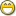### Re: orxEngine Structs

Icy_Viking said...

I just want to make sure I am doing this right.

Well... good news is, you've come to the right place.Icy_Viking said...

orxVector C struct

(snipped)

In C structs, a union indicates values that all take up the same point in memory. So all the first values of the first union (fX,fRho,fR,fH) offset 0. The size of a union is always the size of the largest member, so since each union is only four bytes wide since they each contain only float types. This is all just a very "clever" way to store vectors using different names, depending on the context (plane, sphere, etc.) and personally I'd have written like this, which as a lot easier to understand:

```typedef union __orxVECTOR_t
{
/** Coordinates : 12 */
struct {
orxFLOAT fX;     /**< First coordinate in the cartesian space */
orxFLOAT fY;     /**< Second coordinate in the cartesian space */
orxFLOAT fZ;     /**< Third coordinate in the cartesian space */
} cartesian;
struct {
orxFLOAT fRho;   /**< First coordinate in the spherical space */
orxFLOAT fTheta; /**< Second coordinate in the spherical space */
orxFLOAT fPhi;   /**< Third coordinate in the spherical space */
} spherical;
struct {
orxFLOAT fR;     /**< First coordinate in the RGB color space */
orxFLOAT fG;     /**< Second coordinate in the RGB color space */
orxFLOAT fB;     /**< Third coordinate in the RGB color space */
} rgb;
struct {
orxFLOAT fH;     /**< First coordinate in the HSL color space */
orxFLOAT fS;     /**< Second coordinate in the HSL color space */
orxFLOAT fL;     /**< Third coordinate in the HSL color space */
} hsl;
struct {
orxFLOAT fH;     /**< First coordinate in the HSV color space */
orxFLOAT fS;     /**< Second coordinate in the HSV color space */
orxFLOAT fV;     /**< Third coordinate in the HSV color space */
} hsv;
} orxVECTOR;
```

Icy_Viking said...

Eu code (snipped)

So with everything I said above, what you had is incorrect. The good news is, it's even simpler that what you had. Basically each struct member is at offset 0, 4, or 8 (because floats are always 4 bytes).

```public constant
-- union {
orxVECTOR__fX     =  0, /**< First coordinate in the cartesian space */
orxVECTOR__fRho   =  0, /**< First coordinate in the spherical space */
orxVECTOR__fR     =  0, /**< First coordinate in the RGB color space */
orxVECTOR__fH     =  0, /**< First coordinate in the HSL/HSV color spaces */
-- }
-- union {
orxVECTOR__fY     =  4, /**< Second coordinate in the cartesian space */
orxVECTOR__fTheta =  4, /**< Second coordinate in the spherical space */
orxVECTOR__fG     =  4, /**< Second coordinate in the RGB color space */
orxVECTOR__fS     =  4, /**< Second coordinate in the HSL/HSV color spaces */
-- }
-- union {
orxVECTOR__fZ     =  8, /**< Third coordinate in the cartesian space */
orxVECTOR__fPhi   =  8, /**< Third coordinate in the spherical space */
orxVECTOR__fB     =  8, /**< Third coordinate in the RGB color space */
orxVECTOR__fL     =  8, /**< Third coordinate in the HSL color space */
orxVECTOR__fV     =  8, /**< Third coordinate in the HSV color space */
-- }
SIZEOF_ORXVECTOR  = 12
```
Icy_Viking said...
```public enum orxVECTOR
```

Is this supposed to be a type? I would just declare it as an atom:

```public type orxVECTOR( atom x )
return 1
end type
```
Icy_Viking said...
```public constant C_orxVECTOR = {
```

(snipped)

I don't think you need any of this. That trick is only necessary when you have to pass structs by value but so far I've only found orxVECTOR being passed by pointer. If you want to use an alias for each struct then just C_ORXVECTOR = C_POINTER and be done with it.

-Greg